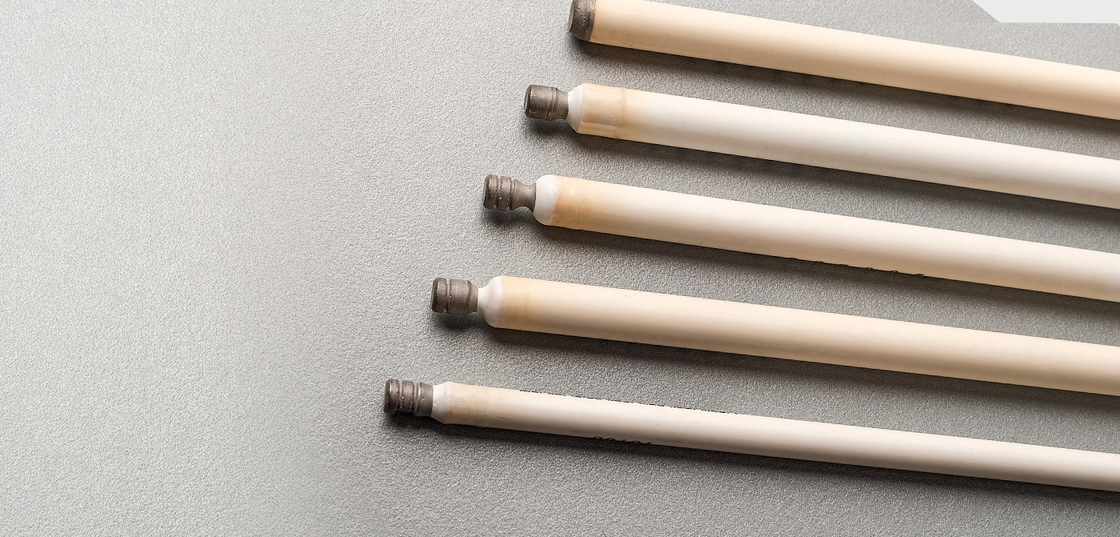83 Wood Street, California Gully

Victoria 3556, Australia

Phone: +61 3 5446 8151

Fax: +61 3 5446 1215>

# Nernst Calculators

The Nernst equation is an equation that relates the reduction potential of an electrochemical reaction (half cell or full cell reaction) to the standard electrode potential. In this application, the Nernst equation is used to calculate the partial pressure (concentration) of oxygen. The equation relies upon temperature and mV reading to provide the oxygen concentration with respect to the reference air concentration (20.9%).

### The Nernst Equation

$$E=\frac{RT}{4F}ln\left(\frac{pO_{2}}{pO'_{2}}\right)$$ Where: E = sensor electromotive force, (mV) R = gas constant T = temperature, (Kelvin) n = number of charges per reactant species F = Faraday constant p = partial pressure (mole fraction) 

The calculators below will allow you to calculate the oxygen concentration from the SIRO2 sensor signal, or the mV for a given oxygen concentration.

### Concentration Calculator

Please enter the operating temperature (500 - 1700 °C) and the measured mV (0 - 1200 mV) signal. The corresponding oxygen concentration is calculated. If it is very low, it is displayed in scientific notation.

If oxygen is to be measured, using a zirconia ceramic electrolyte and atmospheric air, with pO2 = 0.209, as a reference, this equation simplifies to:
pO'2 = 0.209 exp(-46.421 E/T)

Temperature:

Output (mV):

Oxygen:

### Nernst mV Calculator.

The equation may be re-arranged, to allow calculation of the emf from a known reference concentration and a measured oxygen concentration:
E = 0.0496 T log(pO2/0.209 )

This calculator will allow the calculation of the expected Nernst voltage given specific temperature and oxygen values. Note that the oxygen concentration can be entered either as a decimal fraction (so that 1% would be entered as 0.01) or in power of 10 notation ( so that 0.001% would be 1.00*10^-5).

Temperature:

Oxygen:

Output (mV):

Australian Oxytrol Systems supplies a range of industrial, scientific and pottery probes for the measurement of oxygen at high temperature.